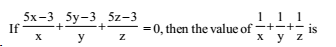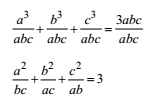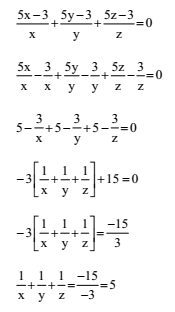# RRB ALP 2018 Practice Test Papers | Arithmetic Questions (Day-40)

Dear Aspirants, Here we have given the Important RRB ALP & Technicians Exam 2018 Practice Test Papers. Candidates those who are preparing for RRB ALP 2018 can practice these Arithmetic Questions to get more confidence to Crack RRB 2018 Examination.

[WpProQuiz 2174]

Click “Start Quiz” to attend these Questions and view Explanation

1. The average height of 8 students is 152 cm. Two more students of heights 144 cm and 155 cm join the group. What is the new average height?

(a) 151.5 cm

(b) 152.5 cm

(c) 151 cm

(d) 150.5 cm

1. A boy found that the average of 20 numbers is 35 when he writes a number ’61’ instead of ’16’. The correct average of 20 numbers is

(a) 32.75

(b) 37.25

(c) 34.75

(d) 34.25

1. The price of rice has increased by 60%. In order to restore the original price, the new price must be reduced by

(a) 33 1/3 %

(b)  371/2 %

(c) 40%

(d) 45%

1. A watch is sold at a profit of 30%. Had it been sold for Rs. 80 less, there would have been a loss of 10%. What is the cost price of rupees?

(a) 150

(b) 200

(c) 400

(d) 800

1. The volume of air in a room is 204 m3. The height of the room is 6 m. What is the floor area of the room?

(a) 34 m2

(b) 32 m2

(c) 46 m2

(d) 44 m2

1. If a+b+c = 0, the value of a2/bc+ b2/ca+ c2/abis

(a) 2

(b) 3

(c) 4

(d) 5

1. A can do a work in 20 days and B can do the same work in 30 days. In how many days can A and B together do the work?

(a) 15

(b) 16

(c) 10

(d) 12

1. If a train maintains an average speed of 42 km per hour, it arrives at its destination at the right time, if however, the average speed is 40 km per hour, it arrives 15 minutes late. Find the length of the journey?

(a) 210 km

(b) 205 km

(c) 209 km

(d) 200 km

1. Prabhat look a certain amount as a loan from a bank at the rate of 8% p.a. simple interest and gave the same amount to Ashish as a loan at the rate of 12% p.a. If at the end of 12 years, he made a profit of Rs. 960 in the deal, then the original amount was:

(a) Rs. 3356

(b) Rs. 1000

(c) Rs. 2000

(d) Rs. 3000

10.(a) 15

(b) 3

(c) 5

(d) 10

Total height of 8 students = 8 × 152 cm = 1216 cm

Total height of 10 students = 1216 cm + 144 cm + 155 cm = 1515 cm

new average = 1515 cm/10= 151.5cm

Sum of 20 numbers = 20 × 35 = 700

Sum of 20 numbers when 61 is replace by 16 => 700 – 61 + 16 = 655

Correct average of 20 numbers = 655/20 = 32.75Difference of P% and L% = 30 – (– 10)= 40%

40/100 x= 80=> x = 200

Volume of air in room = 204 m3

Area of floor × height of room = 204 m3

Area of floor ×6 = 204 m3

Area of floor = 204/6 = 34 m2

If a + b + c = 0 then a3 + b3 + c3 = 3abc

Dividing both sides by abc

A’s 1 day’s work = 1/20

B’s 1 day’s work = 1/30

(A + B)’s 1 day’s work = (1/20+1/30)=5/60

Both A and B will finish the work in 60/5 = 12 days.

Let Length of Journey = X km.

According to question, X/40- X/42= 15/60

X=840/4=210 km

Difference of rates = 4%

Principal xTimexRate/100= 960

Xx12x 4/100 = 960 =>= 960

=> X= 2000RRB ALP 2018 Practice Test Papers | Arithmetic Questions (Day-1)

RRB ALP 2018 Practice Test Papers | Arithmetic Questions (Day-2)

RRB ALP 2018 Practice Test Papers | Arithmetic Questions (Day-3)

RRB ALP 2018 Practice Test Papers | Arithmetic Questions (Day-4)

RRB ALP 2018 Practice Test Papers | Arithmetic Questions (Day-5)

RRB ALP 2018 Practice Test Papers | Arithmetic Questions (Day-6)

RRB ALP 2018 Practice Test Papers | Arithmetic Questions (Day-7)

RRB ALP 2018 Practice Test Papers | Arithmetic Questions (Day-8)

RRB ALP 2018 Practice Test Papers | Arithmetic Questions (Day-9)

RRB ALP 2018 Practice Test Papers | Arithmetic Questions (Day-10)

RRB ALP 2018 Practice Test Papers | Arithmetic Questions (Day-11)

RRB ALP 2018 Practice Test Papers | Arithmetic Questions (Day-12)

RRB ALP 2018 Practice Test Papers | Arithmetic Questions (Day-13)

RRB ALP 2018 Practice Test Papers | Arithmetic Questions (Day-14)

RRB ALP 2018 Practice Test Papers | Arithmetic Questions (Day-15)

RRB ALP 2018 Practice Test Papers | Arithmetic Questions (Day-16)

RRB ALP 2018 Practice Test Papers | Arithmetic Questions (Day-17)

RRB ALP 2018 Practice Test Papers | Arithmetic Questions (Day-18)

RRB ALP 2018 Practice Test Papers | Arithmetic Questions (Day-19)

RRB ALP 2018 Practice Test Papers | Arithmetic Questions (Day-20)

RRB ALP 2018 Practice Test Papers | Arithmetic Questions (Day-21)

RRB ALP 2018 Practice Test Papers | Arithmetic Questions (Day-22)

RRB ALP 2018 Practice Test Papers | Arithmetic Questions (Day-23)

RRB ALP 2018 Practice Test Papers | Arithmetic Questions (Day-24)

RRB ALP 2018 Practice Test Papers | Arithmetic Questions (Day-25)

RRB ALP 2018 Practice Test Papers | Arithmetic Questions (Day-26)

RRB ALP 2018 Practice Test Papers | Arithmetic Questions (Day-27)

RRB ALP 2018 Practice Test Papers | Arithmetic Questions (Day-28)

RRB ALP 2018 Practice Test Papers | Arithmetic Questions (Day-29)

RRB ALP 2018 Practice Test Papers | Arithmetic Questions (Day-30)

RRB ALP 2018 Practice Test Papers | Arithmetic Questions (Day-31)

RRB ALP 2018 Practice Test Papers | Arithmetic Questions (Day-32)

RRB ALP 2018 Practice Test Papers | Arithmetic Questions (Day-33)

RRB ALP 2018 Practice Test Papers | Arithmetic Questions (Day-34)

RRB ALP 2018 Practice Test Papers | Arithmetic Questions (Day-35)

RRB ALP 2018 Practice Test Papers | Arithmetic Questions (Day-36)

RRB ALP 2018 Practice Test Papers | Arithmetic Questions (Day-37)

RRB ALP 2018 Practice Test Papers | Arithmetic Questions (Day-38)

RRB ALP 2018 Practice Test Papers | Arithmetic Questions (Day-39)

RRB ALP 2018 Practice Test Papers | Arithmetic Questions (Day-40)